### 前言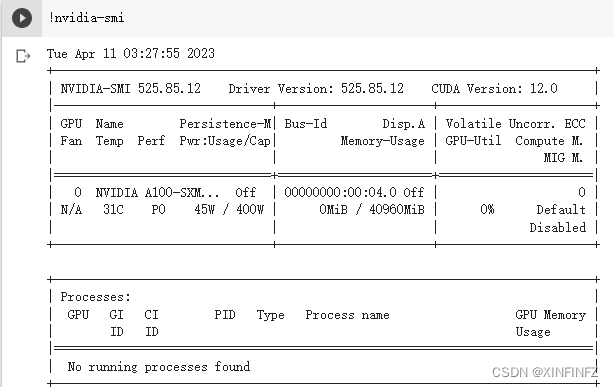### 数据集准备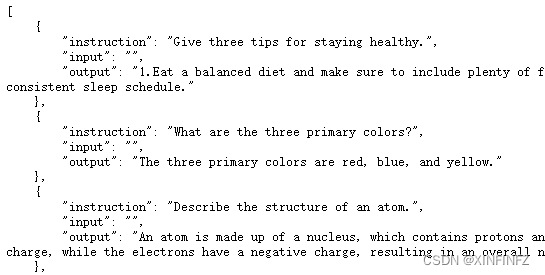``````import json

from datasets import Dataset, DatasetDict

def preprocess_alpaca_json_data(alpaca_dataset_path: str):
instructions = []
inputs = []
outputs = []

for data in alpaca_data:
instructions.append(data["instruction"])
inputs.append(data["input"])
outputs.append(data["output"])

data_dict = {
"train": {"instruction": instructions, "text": inputs, "target": outputs}
}

dataset = DatasetDict()
for k, v in data_dict.items():
dataset[k] = Dataset.from_dict(v)

dataset.save_to_disk(str("./alpaca_data"))
preprocess_alpaca_json_data('你的数据集.json')
``````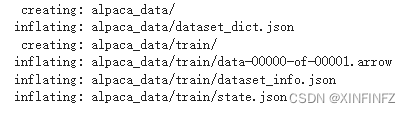### 代码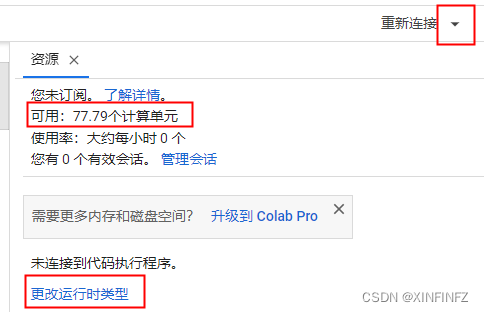``````!pip install accelerate
``````

``````from xturing.datasets.instruction_dataset import InstructionDataset
from xturing.models.base import BaseModel

instruction_dataset = InstructionDataset("/content/alpaca_data")
model = BaseModel.create("bloom_lora")
``````

``````# Finetuned the model
model.finetune(dataset=instruction_dataset)
``````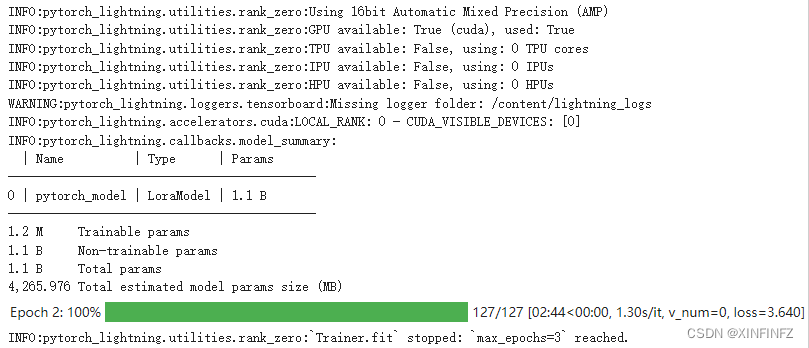``````generation_config = model.generation_config()
generation_config.top_k = 50
generation_config.do_sample = True
generation_config.top_p = 0.8
generation_config.max_new_tokens=512
``````

``````# Once the model has been finetuned, you can start doing inferences
output = model.generate(texts=["第一视角进入风暴中心，是种什么体验？"])
print("Generated output by the model: {}".format(output))
``````

``````登陆后第一时间发现风有多狂，能感觉空气怎么突然就凉爽了。

``````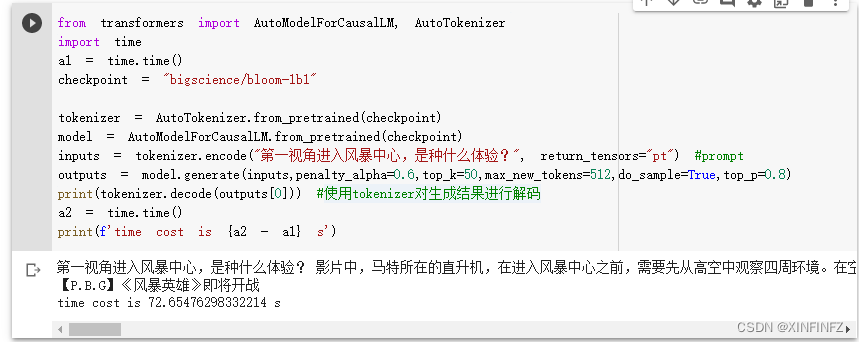``````一视角进入风暴中心，是种什么体验？

``````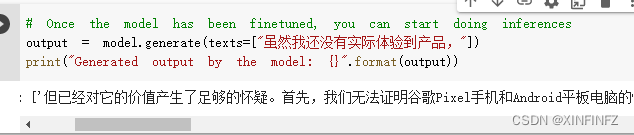``````但已经对它的价值产生了足够的怀疑。

``````

``````虽然我还没有实际体验到产品，所以不敢给一个准确的答案。

``````